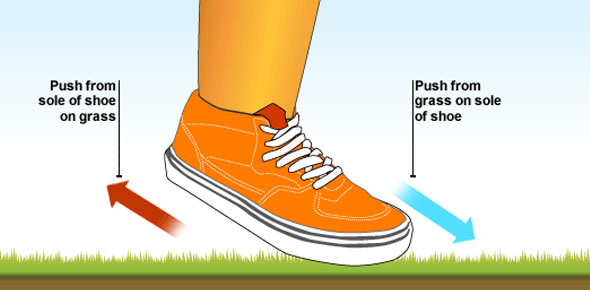# Motion & Forces - Chapter 2

17 Questions | Attempts: 261Settings• 1.
A push or a pull is called
• A.

Motion

• B.

Force

• C.

Acceleration

• D.

Velocity

• 2.
James combines all the forces acting on a tennis ball.  He is finding.....
• A.

Net force

• B.

Inertia force

• C.

Gravitational force

• D.

Frictional force

• 3.
Janet is sliding a box across the floor.  What does she need to know to calculate the force on the box?(F=ma)
• A.

Mass and inertia

• B.

Acceleration and friction

• C.

Mass and acceleration

• D.

Friction and gravity

• 4.
Inertia is the resistance of an object to a change in its
• A.

Gravity

• B.

Motion

• C.

Mass

• D.

Balanced force

• 5.
A soccer player kicks a ball that is at rest.  What do you predict will happen?
• A.

The ball will not move

• B.

The ball will lose momentum

• C.

The ball will accelerate in the direction opposite the applied force

• D.

The ball will accelerate in the same direction as the applied force

• 6.
According to Newton's second law of motion, force equals mass times ......
• A.

Velocity

• B.

Momentum

• C.

Acceleration

• D.

Mass

• 7.
When you push on a door, the door pushes back with an equal and opposite force.  These two forces are known as
• A.

Mass and velocity force pairs

• B.

Unbalanced force pairs

• C.

Mass and acceleration force pairs

• D.

Action/reaction force pairs

• 8.
The momentum of an object is the product of its(p=mv)
• A.

Mass and acceleration

• B.

Mass and velocity

• C.

Force and mass

• D.

Net force and mass

• 9.
A bowling ball rolls down the lane and hits some pins before rolling off the lane.  What happens to the momentum of the ball as it hits the pins?
• A.

Some of the ball's momentum is transferred to the pins

• B.

All of the ball's momentum is transferred to the pins

• C.

None of the ball's momentum is transferred to the pins

• D.

The pins stop the ball from rolling farther

• 10.
Conservation of momentum occurs only when there are........
• A.

No outside forces

• B.

Balanced and unbalanced force pairs

• C.

Gravity and friction

• D.

Mass and inertia

• 11.
What is the unit of force?
• A.

Inch

• B.

Kilometer

• C.

Newton

• D.

Kilogram

• 12.
What symbol is used for force?
• A.

N

• B.

Kg

• C.

F

• D.

Cm

• 13.
What is one of the forces involved in pushing the cart?
• A.

Gravitational force pushing up

• B.

Contact force in pushing

• C.

Inertia force

• D.

Energy force

• 14.
What force is being applied to the cart of it is accelerating at 2.2 m/s2 and the cart's mass is 55 kg?  The formula for calculating force is F=ma
• A.

100N

• B.

25N

• C.

1000N

• D.

121N

• 15.
If you double the force on the cart, what happens to the acceleration?
• A.

It doubles as well

• B.

It gets twice as small

• C.

It triples

• D.

It get ten times bigger

• 16.
What do the data show about momentum as the mass becomes larger? CarabcdefgMass of car (kg)1350140014501500155016001650Momentum (kg m/s)2970308031903300341035203630
• A.

Momentum decreases as mass becomes smaller

• B.

Momentum increases as mass becomes smaller

• C.

Momentum decreases as mass becomes larger

• D.

Momentum increases as mass becomes larger

• 17.
Use the data in the table to determine the velocity of car A.  The formula for calculating velocity is V=P/m (velocity = momentum/mass) carabcdefgmass of car (kg)1350140014501500155016001650momentum (kg m/s)2970308031903300341035203630
• A.

.45 m/s

• B.

2.2 m/s

• C.

50 m/s

• D.

1620 m/s

## Related TopicsBack to top
×

Wait!
Here's an interesting quiz for you.